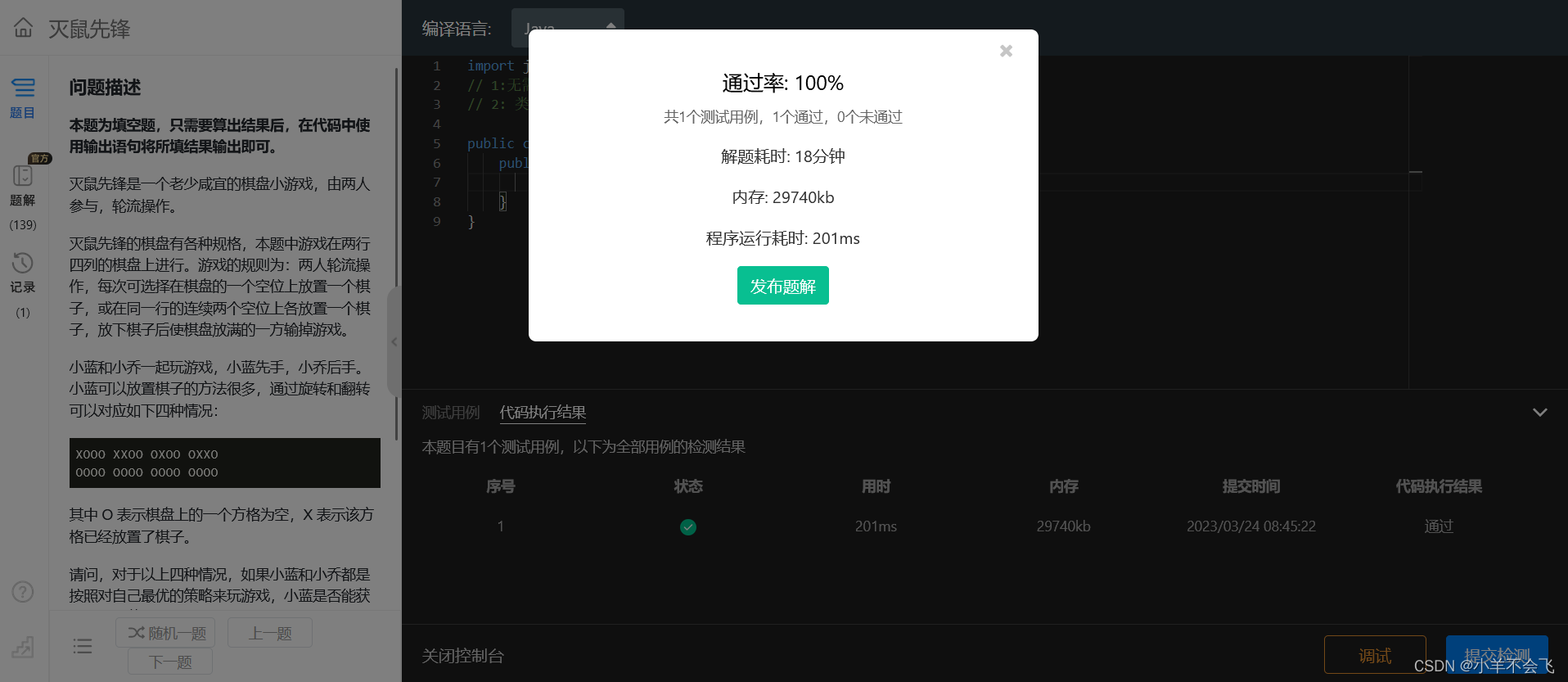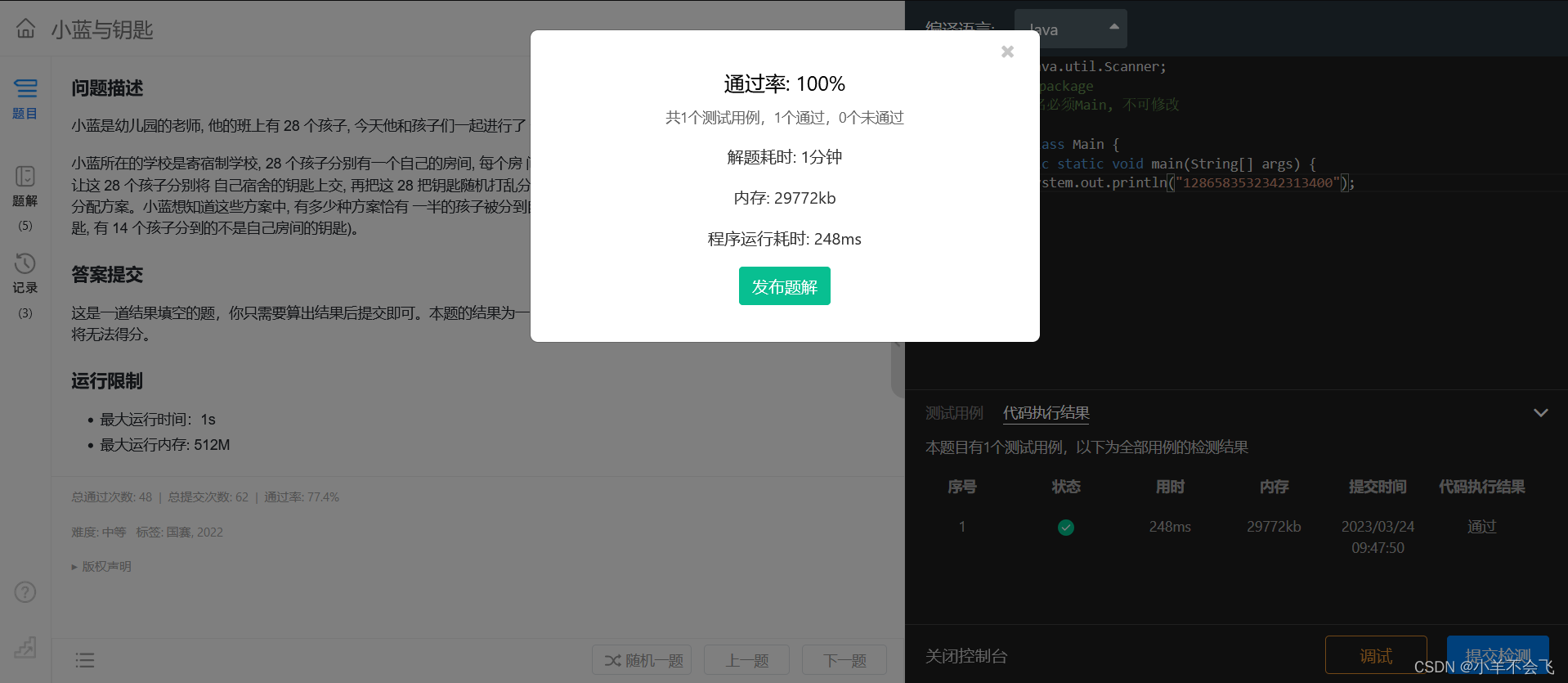Source

# 第 1 题：灭鼠先锋

### 问题描述

``````XOOO XXOO OXOO OXXO
OOOO OOOO OOOO OOOO
``````

• 最大运行时间：1s
• 最大运行内存: 256M

## 代码：

``````package 第十四届蓝桥杯三月真题刷题训练.day21;

/**
* @author yx
* @date 2023-03-24 8:27
*/
public class 灭鼠先锋 {
public static void main(String[] args) {
System.out.println("LLLV");
}
}
``````## 思路：

（1）因为这个题目已经把先手的四种情况进行了说明，所以就非常好想

（2）我们只需要找到一种后手下棋思路让先手必输的情况，那对于后手来说就是最优方法

（3）分别在纸上画一下下棋思路即可

# 第 2 题：小蓝与钥匙

• 最大运行时间：1s
• 最大运行内存: 512M

## 代码：

``````package 第十四届蓝桥杯三月真题刷题训练.day21;

/**
* @author yx
* @date 2023-03-24 8:51
*/
public class 小蓝与钥匙 {
static int N=14;
public static void main(String[] args) {
/**
* 1、先从28个人里面选14个人给定它们的钥匙，一共有C14 28 种选法
* 2、剩下的14个钥匙分别发给不同的人，使他们拿到的都不是自己的钥匙
* 3、后半部分属于全错排列问题，用递推来做
*/
/*
全错排列问题：f(x)=f(x-1)+f(x-2)
f(0)=0;
f(1)=0;
f(2)=1;
*/
//排列组合:2006329977
long C_14_28=paiLie();
long f1=0;
long f2=1;
long temp=0;
for (int i = 3; i <= 14; i++) {
temp=f2;
f2=(i-1)*(f1+f2);
f1=temp;
}
System.out.println(f2*C_14_28);
}

//
static long paiLie(){
long ans=1;
for (long i = 0; i < 14; i++) {
ans=ans*(28-i)/(i+1);
}
return ans;
}
}
``````## 思路 :

（1）先从28个人里面选14个人给定它们的钥匙，一共有C14 28 种选法

``````    static long paiLie(){
long ans=1;
for (long i = 0; i < 14; i++) {
ans=ans*(28-i)/(i+1);
}
return ans;
}``````

（2）剩下的14个钥匙分别发给不同的人，使他们拿到的都不是自己的钥匙，即全错排问题

（3）全错排公式：f(x)=(N-1) * [f(x-1)+f(x-2)]

（4）推导过程：

• 首先是初始值：f1=0; f2=1; f3=9这几项是可以自己手撸的
• 当i>3，很明显手撸不太行，我们静下心来分析，要找其内在的规律
• 设N个人为a,b,c,d...,N张卡为A,B,C,D...
• 若a拿b的卡B,b也拿a的卡A,则显然只剩下N-2个人拿卡,自然是f(N-2)种了(好理解)
• 若a拿b的卡B,b没拿a的卡A,则显然与N-1个人拿卡问题一样,自然是f(N-1)种了(不好理解)
• 为啥是f(N-1)种呢？注意：这里的b没拿卡A，就相当于在N个数中a没拿卡A一样的道理,在N-1个数字中，b的卡片B被a拿走了，而B又不能拿A，其实就是把卡A变相看作是卡B的平替那是不是就相当于看作了N-1个数字进行错排
• a不一定拿B,只要是B,C,D...(N-1个)中的一个就可以了,所以在f(N-1)+f(N-2)再乘上N-1就行了.
• 得出递推公式：f(N)=(N-1)*[f(N-1)+f(N-2)]